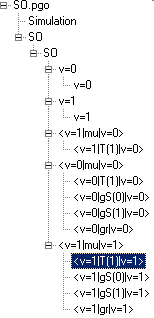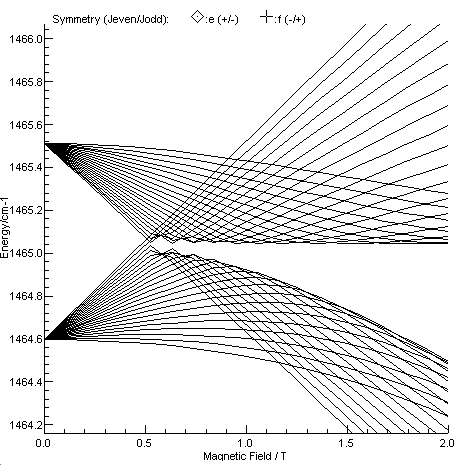Molecule Types Linear Molecules Samples

# Zeeman and Stark Effects in SO

Data file: so.pgo
This example reproduces the results of "Laser magnetic resonance spectroscopy of SO in the X3Σ - state with a CO2 laser as a source", K. Kawaguchi, C. Yamada and E. Hirota, J. Chem. Phys. 71, 3338 (1979) and "Microwave spectrum of the SO radical", F. X. Powell and D. R. Lide, Jr., J. Chem. Phys., 41, 1413 (1964). It provides a good example of calculating the effects of external fields.

The later paper gives rotational constants for both v=0 and v=1 in the ground electronic state, and these are straightforward to enter, as described in Making a Linear Molecule Data File. In this B, λ, γ, DN and λN are available. Following the procedure will produce a simulation of the infra-red spectrum of the fundamental band of SO. Simulating the Zeeman or Stark effect is more complicated, and the complete data file has 3 Transition Moments objects with 9  individual Transition Moment objects beneath them, as summarized in the diagram:The transition moments are made up as follows:

• The <v=1|mu|v=0> Transition Moments object connects the v=1 and v=0 manifolds, and is required to simulate the vibrational transition
• The <v=1|T(1)|v=0> spherical tensor transition moment provides the intensity of the transition, and indicates that it is a standard parallel electric dipole transition.
• The <v=0|mu|v=0> Transition Moments object collects the various dipole moments of the v=0 state, and is required to simulate the microwave spectrum, and the Zeeman and Stark effects.
• The <v=0|T(1)|v=0> spherical tensor transition moment provides the electric dipole moment of v=0, and is essential for the microwave spectrum and the Stark effect.
• The <v=0|gS(0)|v=0> and <v=0|gS(1)|v=0> Spin Magnetic Moments provide the bulk of the magnetic interaction in v=0. In principle this reflects the gSμBB.S interaction between the magnetic field and the electron spin magnetic moment, and leaving the Strength of both at their default values of the g factor of the electron would simulate exactly that. In practice the effective g factor along the axis, <v=0|gS(0)|v=0>, and perpendicular to the axis, <v=0|gS(1)|v=0>, are found to be slightly different. In the Kawasaki et al paper these are expressed as ges + gel = <v=0|gS(1)|v=0> and gel = <v=0|gS(1)|v=0> - <v=0|gS(0)|v=0>. These will also result in magnetic dipole transitions, with different selection rules than electric dipole transitions.
• The <v=0|gr|v=0> rotational magnetic moment is also included for completeness, though here it only has a small effect. Note that PGOPHER expresses this in terms of the nuclear magneton, while Kawasaki et al use the Bohr magneton, so the values differ by mp/me.
•  The <v=1|mu|v=1> Transition Moments object collects the various dipole moments of the v=1 state, and is required to simulate the microwave transitions within v=1, and the Zeeman and Stark effects.of infrared transitions. The transition moment objects beneath it are essentially the same as v=0.

The 32S18O isotope has not been included in the data file, but could be by duplicating the structure above. In principle the <v=1|mu|v=0> Transition Moments object could also have magnetic dipole components, but these are likely to give much weaker transitions, and would only be important in the absence of an electric dipole moment, as in O2.

## Zeeman Plot

Figure 3 of Kawaguchi et al, showing the Zeeman effect in J = 20 and 22, N = 21 in v=1 is re-simulated below.## Line position fitting in the presence of a field

To fit line position data in the presence of a field requires the use of EField and BField directives in the input file, typically immediately before before the affected lines. For example, the data in Table 1 of Kawaguchi at al can be used as follows:

LMR transitions; these are unusual in that the line position is constant, but the field value varies. There are various ways of specifying the data; taking the first transition in the table as an example:

12C18O2 line R(34) 1104.91097 cm-1 N,J = 17,18 ← 18,18 MJ = 18 ← 18 Field = 3004

Right clicking on a simulated peak and copying the line from the linelist window yields:

`BField 0.3004SO v=1  18  -  1 v=0  18  -  3    1104.91097 - 1.8e-7      0 : pQ12(18)18,18 : v=1 v=1 18 17 F1e 18 - v=0 v=0 18 18 F2f 18`

The molecule need not be specified, as there is only one, and manifolds will default to v=1 for the upper state and v = 0 for the lower state so this can be shortened to:

`BField 0.300418 - 1 18 - 3 1104.91097 1`

In this mode the text after the (relative) standard deviation (here 1) is just taken as a comment, so can be omitted, and the symmetry will be ignored in the presence of a field. Only M and the eigenvalue number need to be specified.

The two input lines above require the eigenvalue numbers to be known, which is tricky to work out from the data in the table. Setting the eigenvalue numbers to zero means they will be worked out from the transition label at the end of the line:

`BField 0.300418 - 0 18 - 0 1104.91097 1 : v=1 v=1 18 17 F1e 18 - v=0 v=0 18 18 F2f 18`
The quantum numbers at the end of the line are in order J N and M.

Alternatively branch format can be used:

`BField 0.3004pQ12(18),18,18 1104.91097 1`

The branch is specified in the order:

• p = ΔN = -1
• Q = ΔJ = 0
• 1 = F1 in the upper state (N' = J' - 1)
• 2 = F2 in the lower state (N "= J")
• (18) = Lower state J"
• 18 = Upper state M'
• 18 = Lower state M"
This last format is the easiest when working with published line lists; the complete first block of data will then look like this:
`BField 0.3004pQ12(18)18,18 1104.91097 1BField 0.3213pQ12(18)17,17 1104.91097 1BField 0.3751pQ12(18)15,15 1104.91097 1BField 0.4105pQ12(18)14,14 1104.91097 1`

The last block of data points in the table are also worth commenting on, as these correspond to microwave, rather than infra-red transitions. The field free transitions in v=1 can be input with

`ScaleStdDev 0.1LowerManifold v=1BField 0pR1(0) 0.99900 1rR1(1) 2.09120 1rQ32(1) 0.42537 1rQ32(2) 1.18287 1rQ32(3) 2.16164 1`
Note:
• ScaleStdDev 0.1 implies all the microwave measurements are 10 times more precise. (Alternatively a standard deviation of 0.1 could have been specified for the individual lines.)
• LowerManifold v=1 to override the default lower state.
• BField 0 as these are field free
• The individual lines do not have M specified, as there is no field.
• To display 5 decimal places in the obs-calc values, the Precision needs to be set at the Mixture level.

Finally the v=0 microwave transitions can be input with:

`LowerManifold v=0UpperManifold v=0BField 0.57433pR1(0)-1,0 0.83165 1BField 0.72408rQ32(2)2,2 0.83078 1BField 0.80262rQ32(2)1,2 0.83064 1BField 0.83331rQ32(2)2,1 0.83087 1`